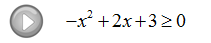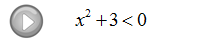## Algebra

Quadratic inequalities can be solved in a number of ways. We will focus on solving them by graphing first and then by using sign charts.  It is important to understand what solutions to these inequalities look like before learning the quick and easy method for solving them.

Method 1: Solve by Graphing

Step 1: Place the inequality in standard form with zero on one side.
Step 2: Graph the quadratic equation.
Step 3: Shade the x-values that produce the desired results.
Step 4: Convert the shading to interval notation.

Solve and express the solution set in interval notation.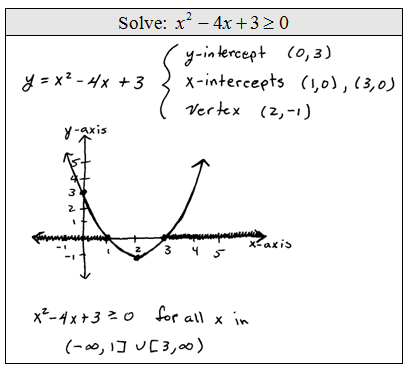In the above example we shaded the x-values for which the graph was above the x-axis. If the problem asked us to solveor for what x-values is the quadratic less than or equal to 0, then the solution would have been all x-values in the interval [1, 3] - the x-values where the graph is below the x-axis. Notice that the numbers on the x-axis x = 1 and x = 3 separate the positive and negative y-values on the graph, these happen to be the x-intercepts.  The x-intercepts or the zeros of a polynomial are called critical numbers.

Solve by graphing.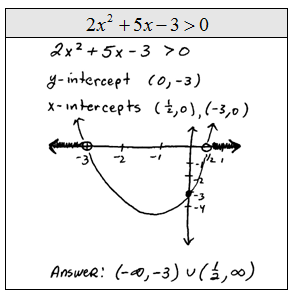Remember to use open dots for strict inequalities < or > and closed dots for inclusive inequalities.

Often it is quite tedious and difficult to graph each inequality when trying to solve them.  There is a shorter method and it involves sign charts.  The idea is to find the critical numbers, the x-values where the y-values could change from positive to negative and create a sign chart to determine which intervals to shade on the x-axis.

Method 2: Solve using sign charts.

Step 1: Place the inequality in standard form with zero on one side.
Step 2: Find the critical numbers (for quadratics - the x-intercepts.)
Step 3: Create a sign chart by determining the sign in each interval bounded by the critical numbers.
Step 4: Use the sign chart to answer the question.

Solve and graph the solution set.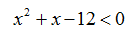Find the critical numbers by setting the quadratic expression equal to zero and solve.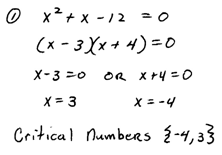Determine the results + or - in each interval bounded by the critical numbers by testing values in each interval.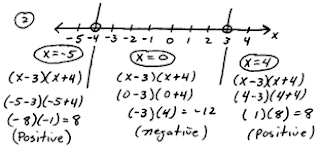Use the sign chart to answer the question. In this case we are looking for the x-values that produce negative results as indicated by the inequality < 0 "less than zero" in the original question.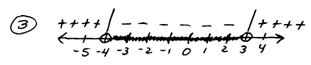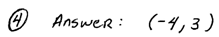When testing values in the intervals created by the critical numbers the actual value is not necessary, we are only concerned with its sign.  The sign + or - will be the same for any value in the interval so you may choose any number within the interval when testing.Solve and graph the solution set.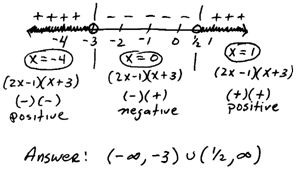Tip: Save some time and just determine if the corresponding y-value is positive or negative. If the polynomial factors then use the factors to determine if the interval will produce positive or negative y-values. There is no need to find the actual values.